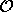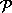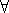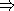## CGAL::Interval_nt<Protected>

### Definition

This section describes briefly what interval arithmetic is, its implementation in CGAL, and its possible use by geometric programs. The main reason for having interval arithmetic in CGAL is its integration into the filtered robust and fast predicates scheme, but we also provide a number type so that you can use it separately if you find any use for it, such as interval analysis, or to represent data with tolerance...

The purpose of interval arithmetic is to provide an efficient way to bound the roundoff errors made by floating point computations. You can choose the behavior of your program depending on these errors. You can find more theoretical information on this topic in [BBP01].

Interval arithmetic is a large concept and we will only consider here a simple arithmetic based on intervals whose bounds are doubles. So each variable is an interval representing any value inside the interval. All arithmetic operations (+, -, *, /, sqrt(), square(), min(), max() and abs()) on intervals preserve the inclusion. This property can be expressed by the following formula (x and y are reals, X and Y are intervals,is an arithmetic operation):xX,yY, (xy)(XY)

For example, if the final result of a sequence of arithmetic operations is an interval that does not contain zero, then you can safely determine its sign.

#include <CGAL/Interval_nt.h>

### Parameters

The template parameter Protected is a Boolean parameter, which defaults to true. It provides a way to select faster computations by avoiding rounding mode switches, at the expense of more care to be taken by the user (see below). The default value, true, is the safe way, and takes care of proper rounding mode changes. When specifying false, the user has to take care about positioning the rounding mode towards plus infinity before doing any computations with the interval class.

FieldWithSqrt

RealEmbeddable

### Types

The class Interval_nt defines the following types:
 typedef double value_type; The type of the bounds of the interval. typedef std::exception unsafe_comparison; The type of the exceptions raised when uncertain comparisons are performed.
 Interval_nt::Protector A type whose default constructor and destructor allow to protect a block of code from FPU rounding modes necessary for the computations with Interval_nt. It does nothing for Interval_nt.

### Creation

 Interval_nt I ( int i); introduces the interval [i;i]. Interval_nt I ( double d); introduces the interval [d;d]. Interval_nt I ( double i, double s); introduces the interval [i;s]. Interval_nt I ( std::pair p); introduces the interval [p.first;p.second].

### Operations

All functions required by a class to be considered as a CGAL number type (see 6) are present, as well as the utility functions, sometimes with a particular semantic which is described below. There are also a few additional functions.

 Interval_nt I / Interval_nt J returns [-;+] when the denominator contains 0. Interval_nt sqrt ( Interval_nt I) returns [0;sqrt(upper_bound(I))] when only the lower bound is negative (expectable case with roundoff errors), and is unspecified when the upper bound also is negative (unexpected case). double to_double ( Interval_nt I) returns the middle of the interval, as a double approximation of the interval. double I.inf () returns the lower bound of the interval. double I.sup () returns the upper bound of the interval. bool I.is_point () returns whether both bounds are equal. bool I.is_same ( Interval_nt J) returns whether both intervals have the same bounds. bool I.do_overlap ( Interval_nt J) returns whether both intervals have a non empty intersection.

The comparison operators (<, >, <=, >=, ==, !=, sign() and compare()) have the following semantic: it is the intuitive one when for all couples of values in both intervals, the comparison is identical (case of non-overlapping intervals). This can be expressed by the following formula (x and y are reals, X and Y are intervals,is a comparison operator):

 (xX,yY, (xy) = true)(XY) = true

and

 (xX,yY, (xy) = false)(XY) =false

Otherwise, the comparison is not safe, and we specify this by returning a type encoding this uncertainty, namely using Uncertain, which can be probed for uncertainty by hand, and which has a conversion to the normal type (e.g. bool) which throws an exception when the conversion is not certain. Note that each failed conversion increments the counter number_of_failures(), and then throw the exception of type unsafe_comparison.

 Uncertain Interval_nt i < Interval_nt j Uncertain Interval_nt i > Interval_nt j Uncertain Interval_nt i <= Interval_nt j Uncertain Interval_nt i >= Interval_nt j Uncertain Interval_nt i == Interval_nt j Uncertain Interval_nt i != Interval_nt j Uncertain compare ( Interval_nt i, Interval_nt j) Uncertain sign ( Interval_nt i) static unsigned number_of_failures () Returns a global counter incremented at each conversion which thrown an exception. typedef Interval_nt Interval_nt_advanced; This typedef (at namespace CGAL scope) exists for backward compatibility, as well as removing the need to remember the Boolean value for the template parameter.advanced### Implementation

The operations on Interval_nt with the default parameter true, are automatically protected against rounding modes, and are thus slower than those on Interval_nt_advanced, but easier to use. Users that need performance are encouraged to use Interval_nt_advanced instead.

Changing the rounding mode affects all floating point computations, and might cause problems with parts of your code, or external libraries (even CGAL), that expect the rounding mode to be the default (round to the nearest).

We provide two interfaces to change the rounding mode. The first one is to use a protector object whose default constructor and destructor will take care of changing the rounding mode.

The second one is the following detailed set of functions :

 typedef int FPU_CW_t; The type used by the following functions to deal with rounding modes. This is usually an int. void FPU_set_cw ( FPU_CW_t R) sets the rounding mode to R. FPU_CW_t FPU_get_cw ( void) returns the current rounding mode. FPU_CW_t FPU_get_and_set_cw ( FPU_CW_t R) sets the rounding mode to R and returns the old one.

The macros CGAL_FE_TONEAREST, CGAL_FE_TOWARDZERO, CGAL_FE_UPWARD and CGAL_FE_DOWNWARD are the values corresponding to the rounding modes.

### Example

Protecting an area of code that uses operations on the class Interval_nt_advanced can be done in the following way:

```{
Interval_nt_advanced::Protector P;
... // The code to be protected.
}
```

The basic idea is to use the directed rounding modes specified by the IEEE 754 standard, which are implemented by almost all processors nowadays. It states that you have the possibility, concerning the basic floating point operations (+,-,*,/,sqrt()) to specify the rounding mode of each operation instead of using the default, which is set to 'round to the nearest'. This feature allows us to compute easily on intervals. For example, to add the two intervals [a.i;a.s] and [b.i;b.s], compute c.i=a.i+b.i rounded towards minus infinity, and c.s=a.s+b.s rounded towards plus infinity, and the result is the interval [c.i;c.s]. This method can be extended easily to the other operations.

The problem is that we have to change the rounding mode very often, and the functions of the C library doing this operation are slow and not portable. That's why assembly versions are used as often as possible. Another trick is to store the opposite of the lower bound, instead of the lower bound itself, which allows us to never change the rounding mode inside simple operations. Therefore, all basic operations, which are in the class Interval_nt_advanced assume that the rounding mode is set to 'round to infinity', and everything works with this correctly set.

So, if the user needs the speed of Interval_nt_advanced, he must take care of setting the rounding mode to 'round to infinity' before each block of operations on this number type. And if other operations might be affected by this, he must take care to reset it to 'round to the nearest' before they are executed.

Notes:

• On Intel platforms (with any operating system and compiler), due to a misfeature of the floating point unit, which does not handle exactly IEEE compliant operations on doubles, we are forced to use a workaround which slows down the code, but is only useful when the intervals can overflow or underflow. If you know that the intervals will never overflow nor underflow for your code, then you can disable this workaround with the flag CGAL_IA_NO_X86_OVER_UNDER_FLOW_PROTECT. Other platforms are not affected by this flag.
• When optimizing, compilers usually propagate the value of variables when they know it's a constant. This can break the interval routines because the compiler then does some floating point operations on these constants with the default rounding mode, which is wrong. This kind of problem is avoided by stopping constant propagation in the interval routines. However, this solution slows down the code and is rarely useful, so you can disable it by setting the flag CGAL_IA_DONT_STOP_CONSTANT_PROPAGATION.advanced